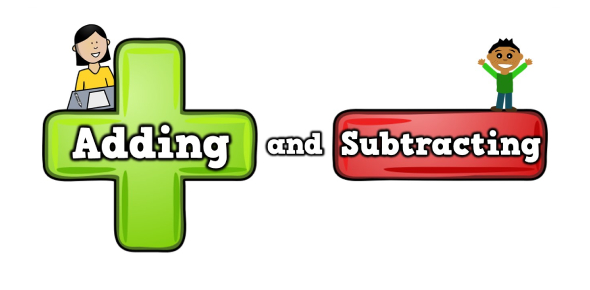# One Step Equations: Addition And Subtraction Quiz

10 Questions | Attempts: 2062
ShareSettings.

• 1.
M+4=-12
• 2.
M-9=-13
• 3.
15+b=23
• 4.
26=8+v
• 5.
V-15=-27
• 6.
3+p=8
• 7.
-15+n=-9
• 8.
X-7=13
• 9.
P-6=-5
• 10.
N+16=9

## Related TopicsBack to top
×

Wait!
Here's an interesting quiz for you.